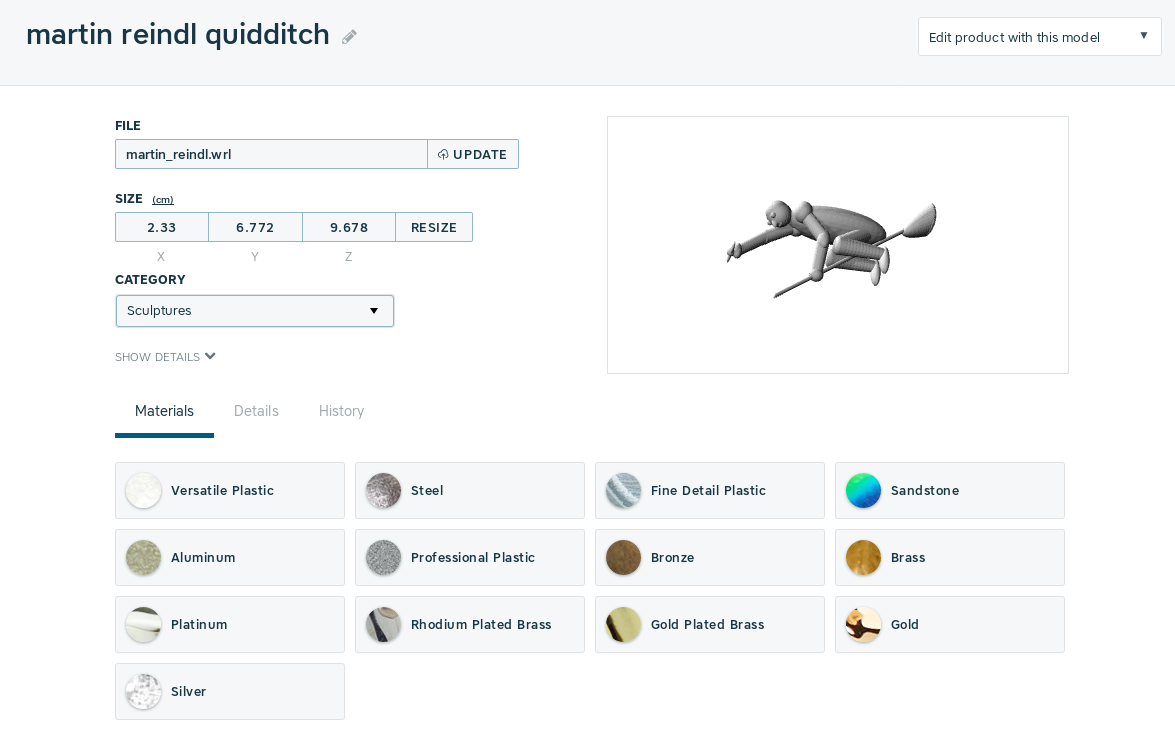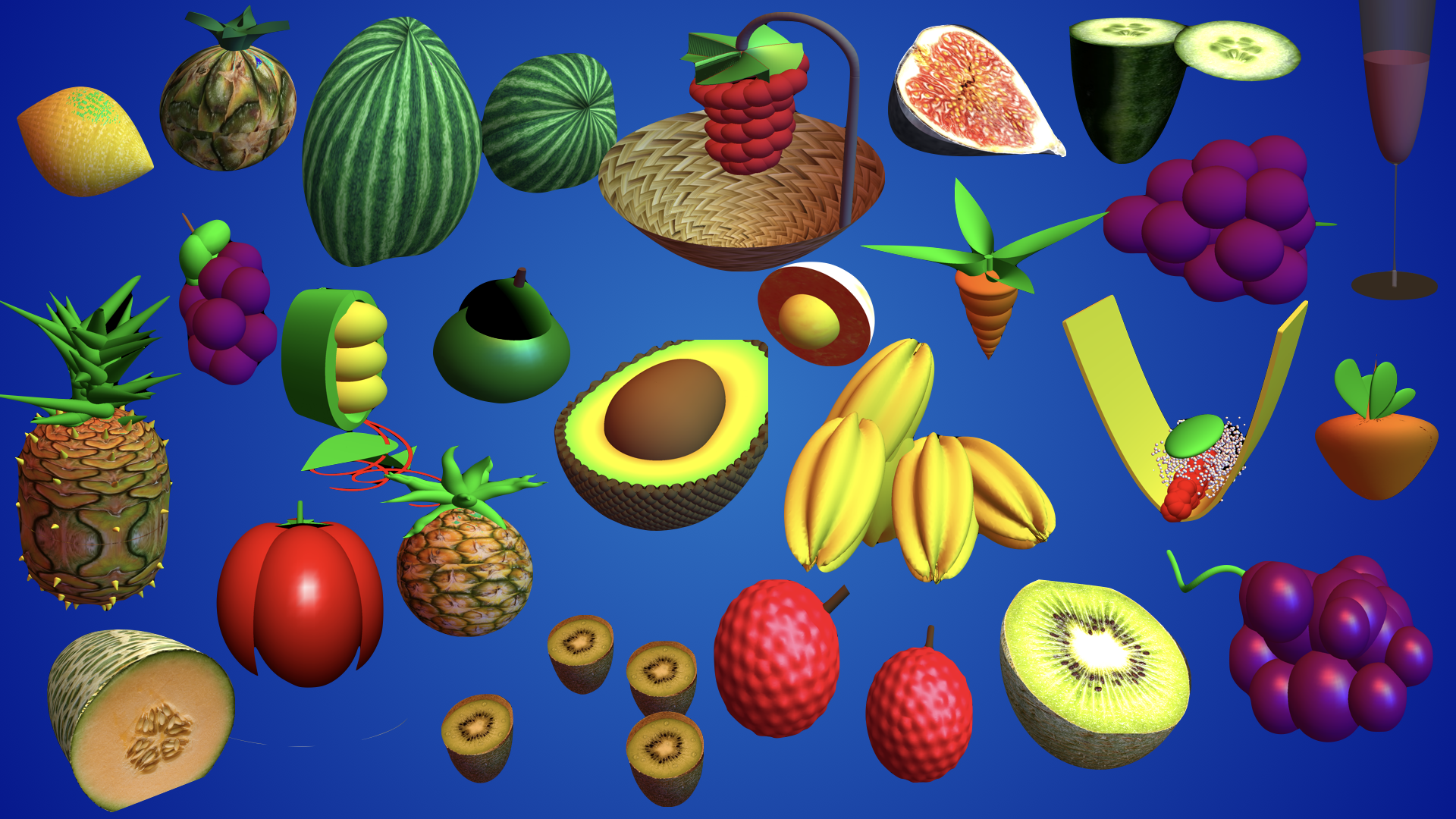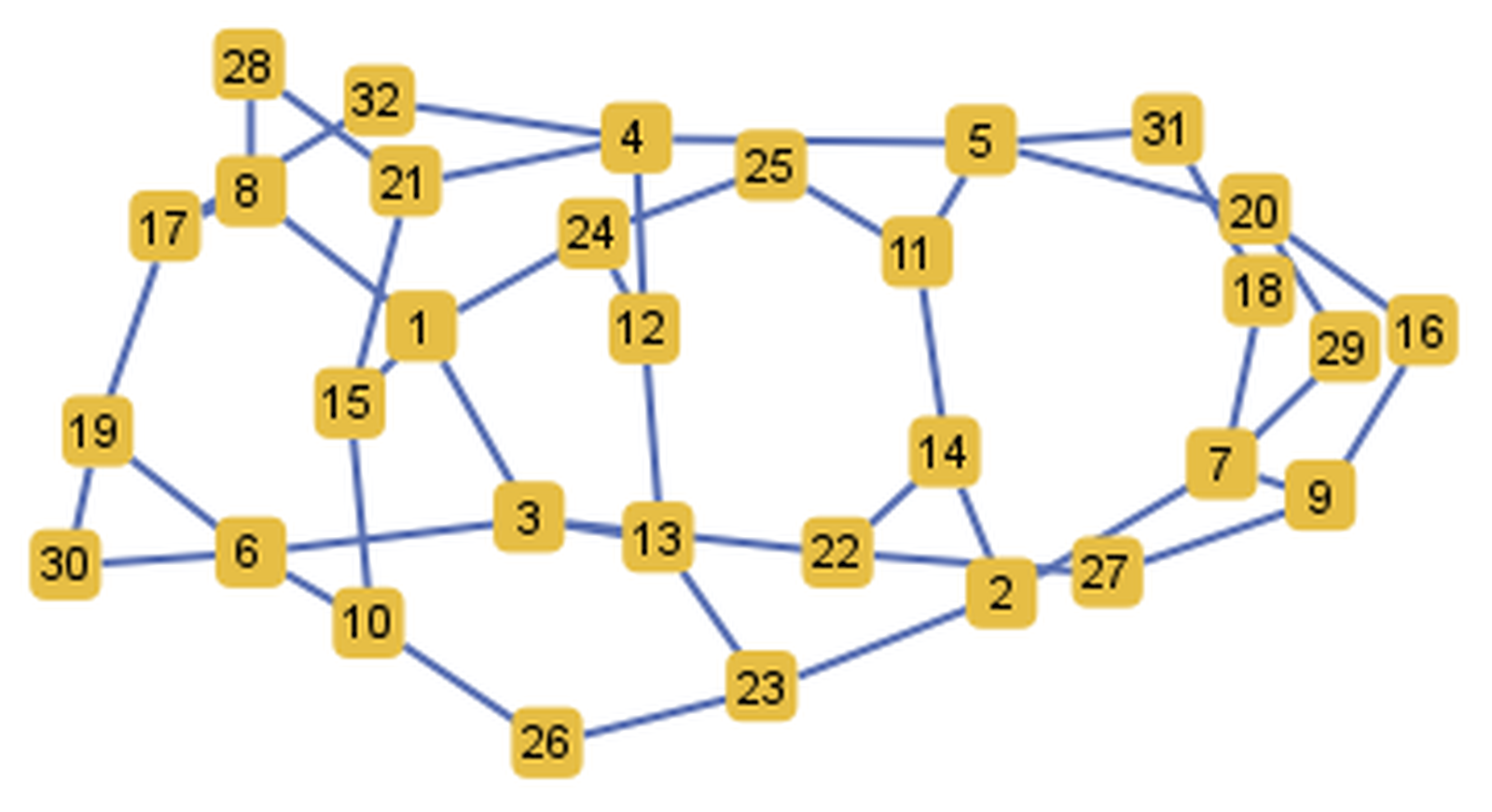# Creativity and computer algebra

Oliver Knill, Harvard University, July 5, 2018

A computer algebra component in a calculus or a linear algebra course is a perfect opportunity to explore creativity and the connections of mathematics with other arts and sciences. It leads to connections of mathematics with other parts. We illustrate this here with Music, Poetry, Sculpture and Puzzles. While many mathematicians perceive Math as part of art itself, the stories can illustrate how the connections can be enhanced with the help of a mathematical computer algebra system. We see that in music, we could generate canons, in poetry find poem prototypes, in sculpture realize mathematical objects and at last in puzzles, see how analyzing puzzles can lead to interesting conjectures.

## Linear algebra and Music

We have had used some music component in the final Mathematica project for a while now in linear algebra courses. Here is an exhibit from 2008. It had been amazing also this year to see the creativity of students. See an exhibit. One can always learn from students. In the workshop, (Here are some slides), I gave the following template for a Canon
```f={1,1,3,3,5,5,1,1,1,1,3,3,5,5,1,1,5,5,6,6,8,8,8,8,5,5,6,6,8,
8,8,8,8,10,8,6,5,5,1,1,8,10,8,6,5,5,1,1,3,3,-4,-4,1,1,1,1};
A = Map[SoundNote, f];
s1 = Sound[{"Piano",              A},     {0, 24}];
s2 = Sound[{"Flute",  RotateRight[A, 8]}, {0, 24}];
s3 = Sound[{"Guitar", RotateRight[A, 16]},{0, 24}];
S = Sound[{s1, s2, s3}, {0, 12}]
Export["brother_john.midi",S,"MIDI"]
Export["brother_john.mp3",S,"MP3"]
```
[MP3] This simple example illustrates how one can join several music 'tracks'. After reading student submissions, I learned that the repetitive part can be avoided by putting "None". Here is a variation, where also the instruments have been replaced:
```n=None; f={1,n,3,n,5,n,1,n,n,n,3,n,5,n,1,n,5,n,6,n,8,n,n,n,5,n,6,n,8,
n,n,n,n,10,8,6,5,n,1,n,8,10,8,6,5,n,1,n,3,n,-4,-4,1,n,1,n};
A  = Map[SoundNote, f];
s1 = Sound[{"Voice", A}, {0, 24}];
s2 = Sound[{"VoiceOohs", RotateRight[A, 8]}, {0, 24}];
s3 = Sound[{"VoiceAahs", RotateRight[A, 16]}, {0, 24}];
s4 = Sound[{"Taiko", A}, {8, 32}];
s5 = Sound[{"Timpani", RotateRight[A, 8]}, {8, 32}];
s6 = Sound[{"Woodblock", RotateRight[A, 16]}, {8, 32}];
S = Sound[{s1, s2, s3, s4, s5, s6}, {0, 10}]
```
[MP3] Is the use of computers to generate music not defeating the process of creativity? One can definitely argue that way, but one can also see the computer as an additional music instrument, as a tool to be creative. Lets try to be creative by applying small changes to the above code. The assignment for the constant n for example could be replaced by a function, which repeats after the period of the canon.
```q:=RandomChoice[{1,3,5,8}];
M=Table[q,{8}]; k=0;n:=Module[{},k++;M[[Mod[k,8]+1]]];
f={1,n,3,n,5,n,1,n,n,n,3,n,5,n,1,n,5,n,6,n,8,n,n,n,5,n,6,n,8,
n,n,n,n,10,8,6,5,n,1,n,8,10,8,6,5,n,1,n,3,n,-4,-4,1,n,1,n};
A  = Map[SoundNote, f];
s1 = Sound[{"Strings", A}, {0, 24}];
s2 = Sound[{"Strings2", RotateRight[A, 8]}, {0, 24}];
s3 = Sound[{"SynthStrings", RotateRight[A, 16]}, {0, 24}];
S = Sound[{s1, s2, s3}, {0, 10}]
```
[MP3] A more sophisticated task is to build three melodies, A,B,C which fit together, then build the canon with structure (A,B,C).

By the way, also in single variable, the connection between math and music is strong. Music can explain some calculus concepts like here: here [PDF]. As part of a HILT project funded by a Hauser grant 2013, we designed some Math 1a lectures of interdisciplanary nature which had been filmed by the Bok center here. Music compositions more closely to calculus already in a in 2007 At that time, the music routines which export midi files were not yet developed in Mathematica, so that the song function was built up.

## Markov chains and Poetry

Music is not the only domain, where one can experiment with art. We have experimented with random poems. Nandy Millan from the University of Birmingham once wrote a Poem generator A.D.A.M. in Java which we translated a couple of years ago in Mathematica: Here is a Mathematica version which we gave to students asking them to modify (like changing the vocabulary or the rules):
```Adjectives = {nice, sad, happy, noisy, cold, beautiful, delicate, shining,
sweet, soft, graceful, pretty, desiring, silly, fragile, silver, golden,
graceful, spiritless, heartbroken, sealed, stormy, rusty, frenetic, burning};
Adverbs = {soon, late, desperately, passionately, slowly, softly, carefully, tenderly,
gently, quickly, kindly};
Articles = {the, a};
Ditverbs = {gave, transformed, told, provided};
Opronouns = {me, you, him, her, us, them};    Spronouns = {I, you, he, she, we};
Itverbs = {raised, felt, anguished, shivered, shined, shouted, whispered, danced, dreamed};
Nouns = {heart, mouth, soul, hand, saint, maiden, love, flower, garden, moon,
sun, bird, breeze, rose, tulip, misery, despair, passion, desire, warmth,
fire, flame, fantasy, wing, kingdom, light, shadow};
Preps = {from, for, with, in} ; Pronouns = {i, you, he, she, it, we, they, me, him, her, us, them};
Tverbs = {loved, hated, wanted, felt, desired, cuddled, greeted, enjoyed};
Verbs = {rose, coped, sang, cried, hoped, shouted, felt, danced, desired, dreamed};
Ditverb := RandomChoice[Ditverbs];     Itverb   := RandomChoice[Itverbs];
Noun := RandomChoice[Nouns];           Opronoun := RandomChoice[Opronouns];
Prep := RandomChoice[Preps];           Pronoun  := RandomChoice[Pronouns];
Spronoun := RandomChoice[Spronouns];   OPronoun := RandomChoice[Opronouns];
Tverb := RandomChoice[Tverbs];         Verb     := RandomChoice[Verbs];
Article = RandomChoice[Articles];
Subject := RandomChoice[{{Article, Noun}, {Spronoun}, {Article, Adjective, Noun}}];
Verblist := {{Itverb}, {Itverb}, {Itverb}, {Tverb}, {Tverb}, {Tverb}, {Tverb}, {Ditverb}};
Predicate := RandomChoice[ Table[{Verblist[[i]], Predicatelist[[i]]}, {i, Length[Verblist]}]];
Object := RandomChoice[{{}, {Adverb}, {Subject}, {Opronoun}, {Prepsubject}}];
Verbobject := {Verb, Object}; Prepsubject := {Prep, Subject}; Subjectpredicate := {Subject, Predicate};
Continuations := If[Length[Object] + Length[Prep] < 4, {"and", Subjectpredicate}, {}];
Sentence := Flatten[RandomChoice[{{Subject, Verbobject}}]];
Verse := Table[Sentence, {5}];
Poem   = Table[Verse,    {3}];
```
Here are some examples. In the following one, where the student Jorma Goerns had used an adaptation of the above Markov procedure in Mathematica to get ideas, then edited to poem so that it rimes:
```He rose passionately
To tackle today's PSET
But then he thought eventually
"Oh, I should have stayed in bed!"

A golden sun was shining
His motivation dying
This function's Fourier series
Remained oh so mysterious!

A deafening cry: Eureka!
Am I not a genius?
He opened Mathematica
And found the Fourier series.
```
There were many other creative approaches. Garrett Tanzer wrote a discrete Markov process which would use a data base of already existing poems to write a new one
```The wide world is wretched.
Speak thy dwelling-place.
Young winter by itself.
The fluttering storm is heaven's hill.
Forgotten for his own element.
They brought her dreams.
In merriment upon the palmy beach.
Sing prison guards at dawn.
And watched the huddled and dolls.
And like ivy over the dawn.
Trembling with surprise. In air.
The sill of the day the present.
Moved in us, hatching marrow.
```
It is sometimes hard to see the difference between actual poems and text generated by a machine.

## Multivariable calculus and Sculpture

In the multivariable calculus part, the 3D printing component has been successful for several years. I learned a lot from Elizabeth Slavkovsky about this who wrote a thesis about the feasability of 3D printing in the classroom See a text. In the fall of 2012 we were able, thanks to an Elson Family arts initiative grant, to print some projects Student submissions. and Some printed species. Here is an example made by Martin Reindl in 2012 and printed in steel. It costed about 70 dollars. In plastic, it would cost 11 dollars.Here are some more recent galleries## Computer science and Puzzles

Computer algebra systems are great to attack puzzles. It can feel a bit of like cheating, but it still requires creativity. It is Creativity of a new kind because one has to be creative to write a program which solves a creative task. Here is a puzzle posed by Anna Beliakova, from the university of Zürich, and Dmitrij Nikolenkov from the High school Trogen in Switzerland:
```Write down the numbers 1-16 in a row so that the sum of two arbitrary
neighbors is a square number.
```
Using mindless force and write all the 20922789888000=16! cases to find the solution would be brutal. More elegant is to define the graph where the numbers 1-16 are the vertices and where two vertices x,y are connected if x+y is a square. Then, we have to find a Hamiltonian path. We can do that by hand as 16 has be a boundary point, forcing the other numbers.
```F[n_]:=Module[{e={}},Do[If[IntegerQ[Sqrt[x+y]],e=Append[e,x->y]],{x,n-1},{y,x+1,n}];e];
Puzzle[n_]:=FindHamiltonianPath[UndirectedGraph[Graph[F[n]]]]
Puzzle
```
The solution is
```{16, 9, 7, 2, 14, 11, 5, 4, 12, 13, 3, 6, 10, 15, 1, 8}
```
We see that 16+9, 9+7, 7+2, 2+14 etc are all squares. Assume now that you are a puzzle writer and want to find out, for which size of puzzles this works.
```Do[If[Length[Puzzle[n]]==0,Print[n]],{n,1,100}]
```
After seeing the output, you might come up with the conjecture that {1,2,5,6,7,8,9,10,11,12,13,14,18,19,20,21,22,24} is the complete list of all these ``square puzzles" which have no solution. I don't know whether this is true. But you see that without the help of a computer, we could hardly have come up with a confident conjecture. An other modification is to ask
```Write down the numbers 1-32 in a circle so that the sum of two arbitrary
neighbors is a square number.
```
Also this defines a graph in which for every edge (a,b), the sum a+b is a square. The task is to find a Hamiltonian cycle in that graph.
```F[n_]:=Module[{e={}},Do[If[IntegerQ[Sqrt[x+y]],
e=Append[e,x->y]],{x,n-1},{y,x+1,n}];e];
Puzzle[n_]:=FindHamiltonianCycle[UndirectedGraph[Graph[F[n]]]]
S=Graph[UndirectedGraph[F],
{FormatType -> TraditionalForm, GraphStyle -> "SmallNetwork"}]
Export["graph.png",S,"PNG",ImageSize->1600]
Puzzle
```
Here is the solution
```{1,15,10,26,23,2,14,22,27,9,16,20,29,7,18,31,5,11,
25,24,12,13,3,6,30,19,17,32,4,21,28,8}
```After running the same experiment for arbitrary puzzles, we can ask that the smallest Hamiltonian circle puzzle has a solution for n=32 and that there is a solution for every n larger or equal than 32. We are not aware that this question has been asked already, nor do we know how hard it would be to prove this.
```Do[If[Length[Puzzle[n]]==0,Print[n]],{n,1,100}]
```
Now, finding a solution to the particular puzzle like for n=1000 would be hard to do without a computer. The solution starts with 1->899 -> 950 -> 11 -> 889 -> 480 ,...

By the way, William Rowan Hamilton, came up with the context of Hamiltonian path when looking at the "icosian game" which asked to find a Hamiltonian cycle in the dodecahedron. Hamilton's puzzle was marketed but never became a commercial success. But it became much more, the concept of Hamiltonian path has enormous impact in mathematics.